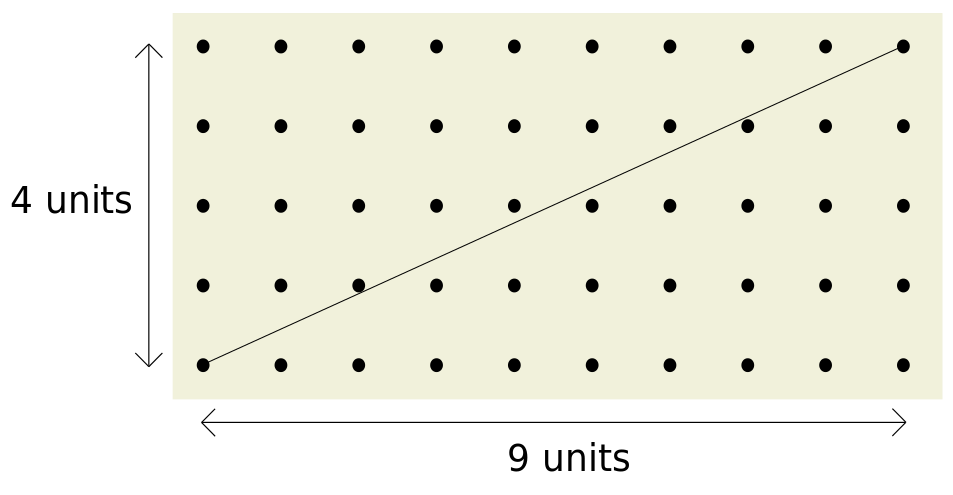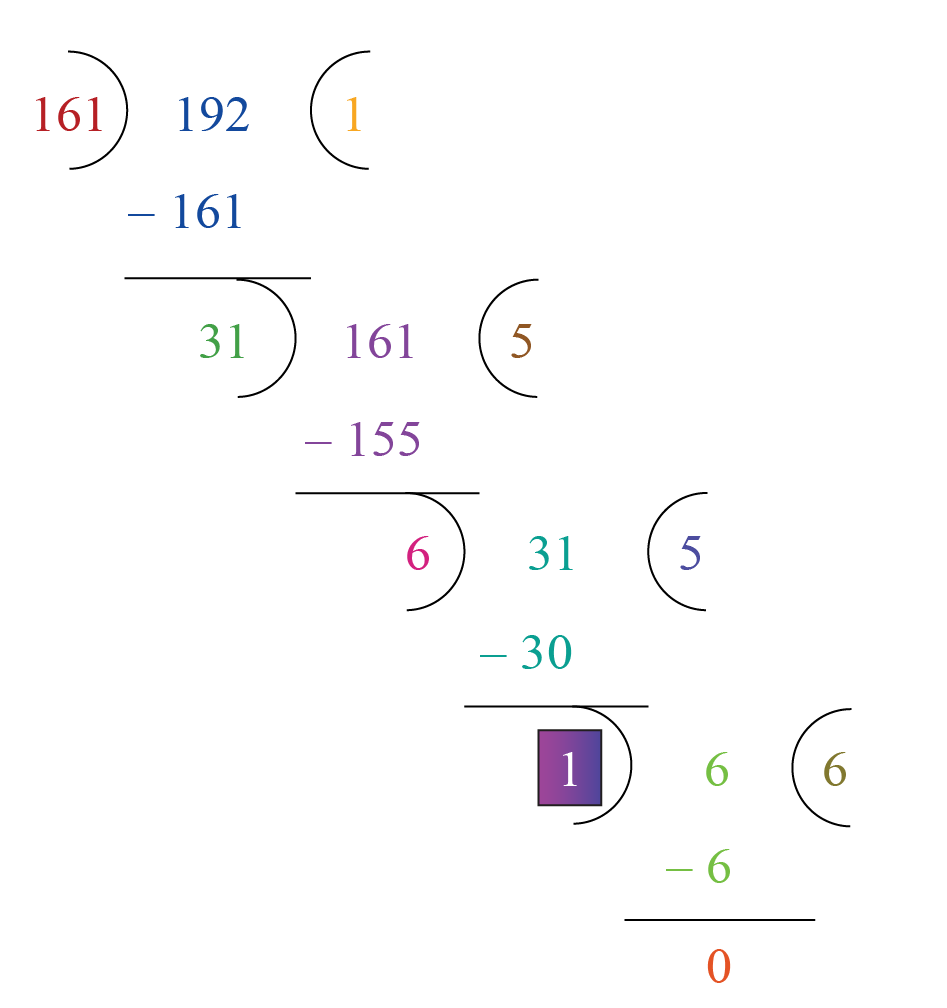Mathematics

# Co-Primes

745 views

 1 Introduction 2 What are Co-Prime Numbers? 3 Euclid’s Algorithm 4 Properties of Co-Primes 5 Co-prime numbers in Number theory 6 Summary and Applications in Real life 7 FAQs

18 September 2020

## Introduction

Co-Prime Numbers are such numbers that have their greatest common divisor equal to unity, i.e. they have no other common divisor apart from one.

There should at least be a pair of numbers to be called Co-Primes.

Let us take the example of $$(4, 9)$$

Factors of $$4: 1, 2, 4$$

Factors of $$9: 1, 3, 9$$

$$\text{GCD (4,9) = (4,9) = 1}$$## What are Co-Prime Numbers?

In Mathematics, any two numbers are co-prime or relatively prime or mutually prime if the only number that divides both of them is unity. This is indeed equivalent to their Greatest Common Divisor (GCD) being 1.

Any fraction is called a reduced fraction if its numerator and denominator are coprime. A fast and efficient way to determine if two numbers are prime or not is by using Euclid’s Algorithm. There are also faster variants of Euclid’s Algorithm known as the Binary GCD Algorithm or Lehmar’s GCD Algorithm.

## Euclid’s Algorithm:

The Euclidean Algorithm is a technique for quickly finding the GCD of two integers.

### The Euclidean Algorithm for finding GCD(A, B) is as follows:

• If $$\text{A} = 0$$ then $$\text{GCD(A,B)=B,}$$ since the $$\text{GCD(0,B)=B,}$$and we can stop.

• If $$\text{B = 0}$$ then $$\text{GCD (A, B)=A,}$$ since the $$\text{GCD (A, 0)=A,}$$ and we can stop.

• Write $$\text{A}$$ in quotient remainder form $$\text{(A = B⋅Q + R)}$$

• Find $$\text{GCD (B, R)}$$ using the Euclidean Algorithm since $$\text{GCD (A, B) = GCD (B, R)}$$

### Implementation:

Implementations of algorithms are often expressed in Psuedocodes. For example, the division-based version may be programmed as

 function gcd(a, b)       while b ≠ 0             t := b             b := a mod b             a := t        return a

For a detailed explanation and understanding of the above algorithm, one can visit :

One can also use the Co-Prime Calculator by Cuemath to check if two numbers are co-prime or not:

https://www.cuemath.com/numbers/coprime-numbers/#Coprime-Numbers-Calculator

## Properties of Co-Primes:

1. Unity is co-prime with every other number.

2. Every prime is co-prime to each other. This can be understood by taking any two different primes $$\text{P1}$$ and $$\text{P2}.$$

Factors of $$\text{P1 : 1, P1}$$

Factors of $$\text{P2: 1, P2}$$

Therefore, $$\text{GCD (P1,P2) = (P1,P2) = 1 (Since P1 ≠ P2)}$$

3. The sum of numbers is always coprime to the product of the numbers.

Consider 2 numbers, x and y. Then,

$$\text{GCD (x+y,x*y) = (x+y,x*y) = 1}$$

4. Any two consecutive numbers are always prime i.e. $$\text{∀ n > 1}$$

$$\text{GCD (n,n+1) = (n,n+1) = 1}$$

5. For any prime $$𝑝$$

$$𝑝$$ has $$𝑝 − 1$$ Co-primes

$$𝑝^2$$ has $$𝑝(𝑝 – 1)$$ Co-primes

$$𝑝^3$$ has $$𝑝^2(𝑝 – 1)$$ Co-primes

$$⋮$$

$$𝑝^𝑘$$ has $$𝑝^{𝑘−1}(𝑝 − 1)$$ Co-primes

In particular, if $$𝑛$$ is a power of $$2,$$ there are $$\frac{𝑛}{2}$$ Co-primes of $$𝑛,$$ and if 𝑛 is a power of $$3,$$ there are $$2\; 3\, 𝑛$$ Co-primes of $$𝑛.$$

6. For a number whose prime factorization is $$𝑝_1^{𝑎1}𝑝_2^{𝑎2} ⋯ 𝑝_𝑘^{𝑎𝑘},$$ the number of Coprimes is

$$𝑝_1^{𝑎1−1} 𝑝_2^{𝑎2−1} ⋯ 𝑝_𝑘^{𝑎𝑘−1} (𝑝1 − 1)(𝑝2 − 1) ⋯ (𝑝𝑘 − 1)$$.

## Co-Primes in Number Theory

In number theory, two integers a and b are said to be relatively prime, mutually prime, or coprime (also written co-prime) if the only positive integer (factor) that divides both of them is 1In number theory, two integers a and b are said to be relatively prime, mutually prime, or coprime (also written co-prime) if the only positive integer (factor) that divides both of them is $$1.$$

The number of integers coprime to a positive integer n, between 1 and n, is given by Euler's totient function, also known as Euler's phi function, $$φ(n).$$

A set of integers can also be called coprime if its elements share no common positive factor except $$1.$$ A stronger condition on a set of integers is pairwise coprime, which means that a and b are coprime for every pair (a, b) of different integers in the set. The set $$\text{{2, 3, 4}}$$ is coprime, but it is not pairwise coprime since $$2$$ and $$4$$ are not relatively prime.

## Solved Examples

 Example 1

Show that $$161$$ and $$192$$ are coprime numbers.

Solution:

We will find the HCF of the given numbers $$161$$ and $$192$$ using the division method.The HCF of $$161$$ and $$192$$ is $$1$$

Thus, they are coprime numbers

 Example 2

If $$53$$ and $$107$$ are coprime, what would be their HCF?

Solution:

It is given that $$53$$ and $$107$$ are coprime.

They cannot have any common factor other than $$1.$$

Hence, their HCF is $$1.$$

 Example 3

If $$23$$ and $$7$$ are coprime numbers, what is their LCM?

Solution:

It is given that $$23$$ and $$7$$ are coprime numbers.

Hence, their LCM is equal to their product,

$$23 \times 7 = 161.$$

## 1. What is the LCM of two numbers?

- LCM of two numbers is defined as the least common multiple of those two numbers, i.e. the smallest number divisible by both the given numbers. From the definition, it is clear that

$$\text{min (A, B) <= LCM (A, B) <= A*B}$$

## 2. What is the GCD of two numbers?

- GCD of two numbers is defined as the Greatest Common Divisor of those two numbers, i.e. the biggest number that can divide both the given numbers.

From the definition it is clear that

$$\text{1 <= GCD (A, B) <= min (A, B)}$$

## 3. What is the relationship between LCM and GCD of two numbers?

- The product of the LCM and GCD of two numbers is indeed the product of the two numbers.

$$\text{LCM (A, B) * GCD (A, B) = A*B}$$

## What is the LCM of two coprime numbers?

As we learned that coprime numbers have their $$\text{GCD} = 1,$$ from the previous formula

$$\text{LCM (A,B) * 1 = A*B}$$

$$\text{LCM (A,B) = A*B}$$

## What are co-prime Numbers?

Co-Prime Numbers are those that have their $$\text{GCD}=1.$$

GIVE YOUR CHILD THE CUEMATH EDGE
Access Personalised Math learning through interactive worksheets, gamified concepts and grade-wise courses